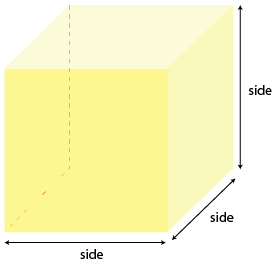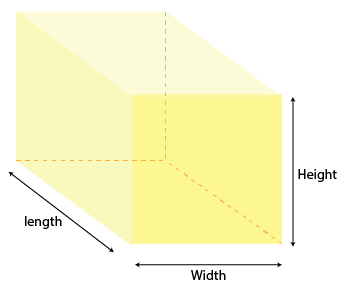Algebraic Identity (a-b)³

## Objective

To verify the algebraic identity (a-b)³=a³-b³-3(a-b)ab

## Algebraic Identity

• An algebraic identity is an equality that is true for all the values of the variables in the equality.
• They are also used for the factorization of polynomials.
• The algebraic equations that are valid for all values of variables in them are called algebraic identities.
• In this way, algebraic identities are used in the computation of algebraic expressions and solving different polynomials.

## Cube

A cube is a three-dimensional solid object bounded by six square faces, It has 6 faces, 12 edges, and 8 vertices. cube have a length of Height, width, and thickness are equal. In the case of Cuboid, these lengths are not equal.## Volume Cube

The volume of the cube is the space contained in it. Suppose, if an object is in cubical shape, and we need to immerse any material in it, say water, then measure the amount of water in litres to be kept in the object is calculated by its volume. The formula for the volume is given by: Volume of the cube= (side)³

## Cuboid

A cuboid is a 3-D shape with sides that are rectangular. Cuboids have six surfaces and twelve edges. Objects that are cuboids include books, matchboxes, and shoeboxes. If a cuboid has faces that are all square, it is a cube. All the angles of the cuboid are right angles.## Volume of Cuboid

The product of the length of each side itself.

Formula : Volume = height x width x thikness

## Volume of Cuboid:

Volume of cuboid is the total number of spaces occupied by the cuboid in a three dimensional space. A cuboid is a three-dimensional structure. having six rectangular faces. These six faces of the cuboid exist as a pair of three parallel faces. Therefore, the volume is a measure of based on the dimensions of these faces, i.e., length, width, and height. Volume of cubiod = length * width * height = l * b * h(cubic unit)

Prerequisite:

Concept of cube

Concept of cuboid

Subtraction of terms

Multipication of terms

## Volume of cube

The product of its height, width, and thickness

Formula : Area = side x side x side = side³